## The Comparison Test

Theorem 6.4.1 (The Comparison Test)   Suppose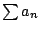and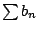are series with all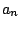and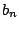positive and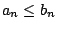for each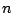.
1. Ifconverges, then so does.
2. Ifdiverges, then so does.

Proof. [Proof Sketch] The condition of the theorem implies that for any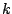,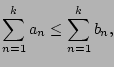from which each claim follows.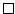Example 6.4.2   Consider the series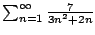. For eachwe have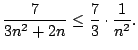Since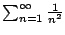converges, Theorem 6.4.1 implies thatalso converges.

Example 6.4.3   Consider the series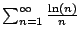. It diverges since for each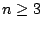we have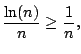and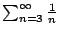diverges.

William Stein 2006-03-15